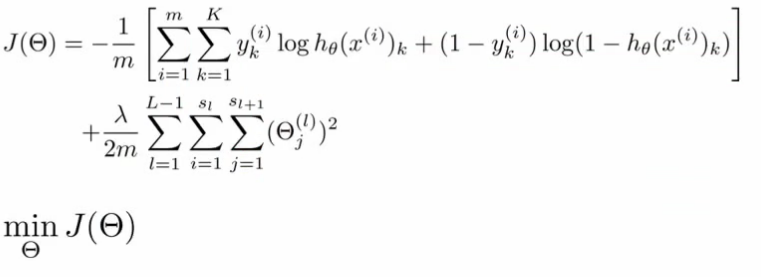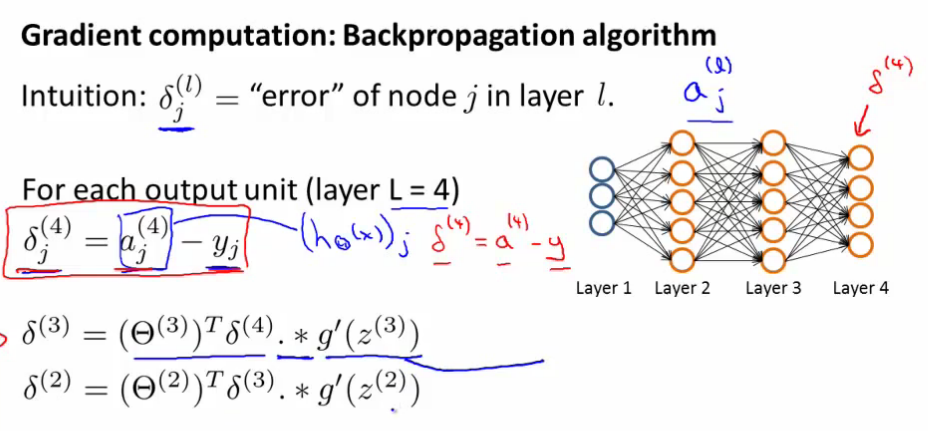swizec.com

# I suck at implementing neural networks in octave

A few days ago I implemented my first full neural network in Octave. Nothing too major, just a three layer network recognising hand-written letters. Even though I finally understood what a neural network is, this was still a cool challenge.

Yes, even despite having so much support from ml-class ... they practically implement everything and just leave the cost and gradient functions up to you to implement. Then again, Octave provides tools for learning where you essentially just run a function, tell it where to find the cost and gradient function and give it some data.

Then the magic happens.

Getting the basic implementation to work is really simple since the formulas being used aren't all that complex:Here's the code I've come up with to get this working on a three layer network:

```function [J grad] = nnCostFunction(nn_params, ...                                   input_layer_size, ...                                   hidden_layer_size, ...                                   num_labels, ...                                   X, y, lambda)%NNCOSTFUNCTION Implements the neural network cost function for a two layer%neural network which performs classification%   [J grad] = NNCOSTFUNCTON(nn_params, hidden_layer_size, num_labels, ...%   X, y, lambda) computes the cost and gradient of the neural network. The%   parameters for the neural network are "unrolled" into the vector%   nn_params and need to be converted back into the weight matrices.%%   The returned parameter grad should be a "unrolled" vector of the%   partial derivatives of the neural network.%.css-13aqjzy{display:inline-block;}
% Reshape nn_params back into the parameters Theta1 and Theta2, the weight matrices% for our 2 layer neural networkTheta1 = reshape(nn_params(1:hidden_layer_size * (input_layer_size + 1)), ...                 hidden_layer_size, (input_layer_size + 1));
Theta2 = reshape(nn_params((1 + (hidden_layer_size * (input_layer_size + 1))):end), ...                 num_labels, (hidden_layer_size + 1));
% Setup some useful variablesm = size(X, 1);
% You need to return the following variables correctlyJ = 0;Theta1_grad = zeros(size(Theta1));Theta2_grad = zeros(size(Theta2));
yy = zeros(size(y),num_labels);for i=1:size(X)  yy(i,y(i)) = 1;end
X = [ones(m,1) X];% costfor  i=1:m  a1 = X(i,:);  z2 = Theta1*a1';  a2 = sigmoid(z2);  z3 = Theta2*[1; a2];  a3 = sigmoid(z3);
J += -yy(i,:)*log(a3)-(1-yy(i,:))*log(1-a3);end
J /= m;
J += (lambda/(2*m))*(sum(sum(Theta1(:,2:end).^2))+sum(sum(Theta2(:,2:end).^2)));
t=1;for t=1:m  % forward pass  a1 = X(t,:);  z2 = Theta1*a1';  a2 = [1; sigmoid(z2)];  z3 = Theta2*a2;  a3 = sigmoid(z3);
% backprop  delta3 = a3-yy(t,:)';  delta2 = (Theta2'*delta3).*[1; sigmoidGradient(z2)];  delta2 = delta2(2:end);
end```

This then basically gets pumped into the fmincg function and on the other end a result pops out.

Now, I've managed to vectorize this thing to the edge of my capabilities. But I know it's still just matrix multiplication so I know for a fact it should be possible to vectorize even further. Anyone know how to do that?

Also, if you know of a cool way to generalize the algorithm so it would work on bigger networks, I'd love to hear about that as well!Published on November 15th, 2011 in Artificial intelligence, Machine learning, Neural network, Octave, Uncategorized,

## Learned something new? Read more Software Engineering Lessons from Production

I write articles with real insight into the career and skills of a modern software engineer. "Raw and honest from the heart!" as one reader described them. Fueled by lessons learned over 20 years of building production code for side-projects, small businesses, and hyper growth startups. Both successful and not.

Subscribe below 👇

### Software Engineering Lessons from Production

Join Swizec's Newsletter and get insightful emails 💌 on mindsets, tactics, and technical skills for your career. Real lessons from building production software. No bullshit.

"Man, love your simple writing! Yours is the only newsletter I open and only blog that I give a fuck to read & scroll till the end. And wow always take away lessons with me. Inspiring! And very relatable. 👌"

Join 15,883+ engineers learning lessons from my "raw and honest from the heart" emails.

⭐️⭐️⭐️⭐️✨
4.5 stars average rating

Have a burning question that you think I can answer? Hit me up on twitter and I'll do my best.

Who am I and who do I help? I'm Swizec Teller and I turn coders into engineers with "Raw and honest from the heart!" writing. No bullshit. Real insights into the career and skills of a modern software engineer.

Want to become a true senior engineer? Take ownership, have autonomy, and be a force multiplier on your team. The Senior Engineer Mindset ebook can help 👉 swizec.com/senior-mindset. These are the shifts in mindset that unlocked my career.

Curious about Serverless and the modern backend? Check out Serverless Handbook, for frontend engineers 👉 ServerlessHandbook.dev

Want to Stop copy pasting D3 examples and create data visualizations of your own? Learn how to build scalable dataviz React components your whole team can understand with React for Data Visualization

Want to get my best emails on JavaScript, React, Serverless, Fullstack Web, or Indie Hacking? Check out swizec.com/collections

Want to brush up on modern JavaScript syntax? Check out my interactive cheatsheet: es6cheatsheet.com

Did someone amazing share this letter with you? Wonderful! You can sign up for my weekly letters for software engineers on their path to greatness, here: swizec.com/blog

Want to brush up on your modern JavaScript syntax? Check out my interactive cheatsheet: es6cheatsheet.com

By the way, just in case no one has told you it yet today: I love and appreciate you for who you are ❤️

Created by Swizec with ❤️